# Prolog and Logic Languages Aaron Bloomfield CS 415

• Slides: 27
Download presentationProlog and Logic Languages Aaron Bloomfield CS 415 Fall 2005 1Prolog lectures • Today – Overview of Prolog – Homework distributed – (also plan on spending time on Ocaml HW) • Next lecture – More specifics of programming prolog • How to install and use on Windows • Gotchas • Etc. 2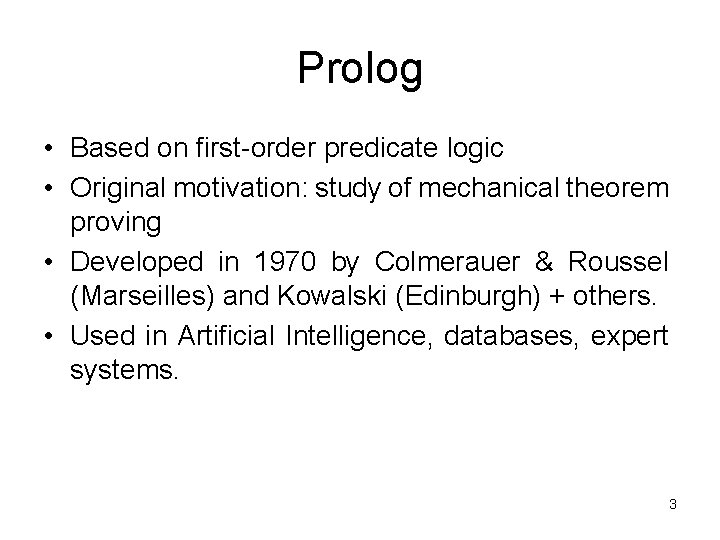Prolog • Based on first-order predicate logic • Original motivation: study of mechanical theorem proving • Developed in 1970 by Colmerauer & Roussel (Marseilles) and Kowalski (Edinburgh) + others. • Used in Artificial Intelligence, databases, expert systems. 3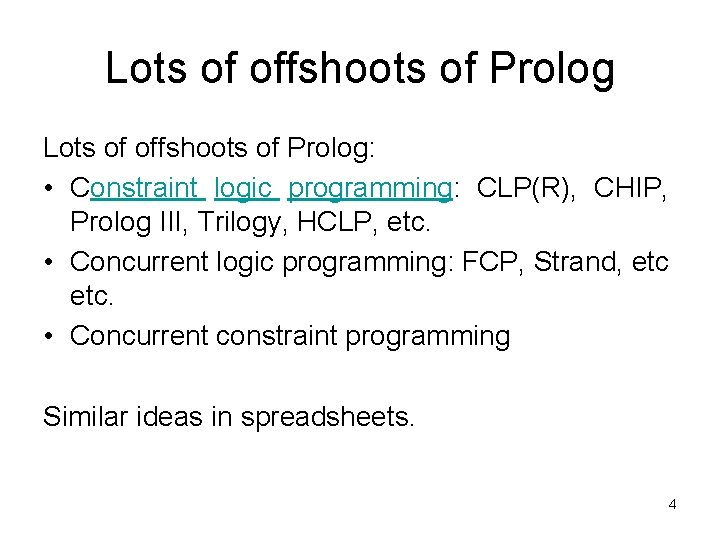Lots of offshoots of Prolog: • Constraint logic programming: CLP(R), CHIP, Prolog III, Trilogy, HCLP, etc. • Concurrent logic programming: FCP, Strand, etc. • Concurrent constraint programming Similar ideas in spreadsheets. 4Prolog Programs • Program = a bunch of axioms • Run your program by: – Enter a series of facts and declarations – Pose a query – System tries to prove your query by finding a series of inference steps • “Philosophically” declarative • Actual implementations are deterministic 5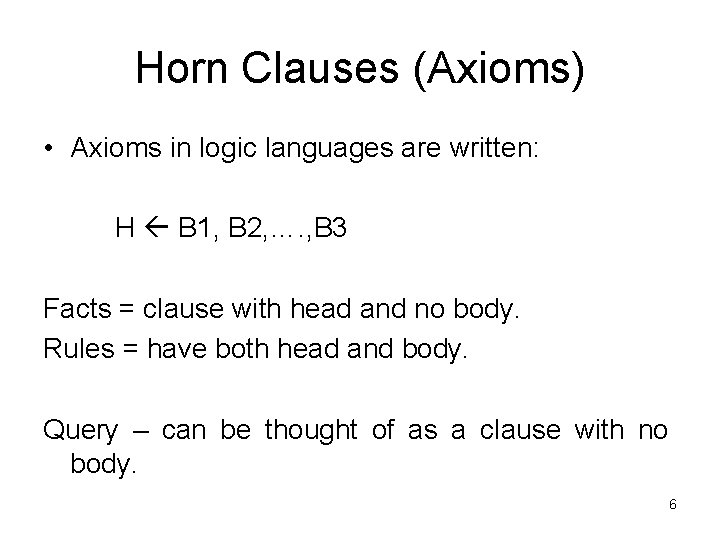Horn Clauses (Axioms) • Axioms in logic languages are written: H B 1, B 2, …. , B 3 Facts = clause with head and no body. Rules = have both head and body. Query – can be thought of as a clause with no body. 6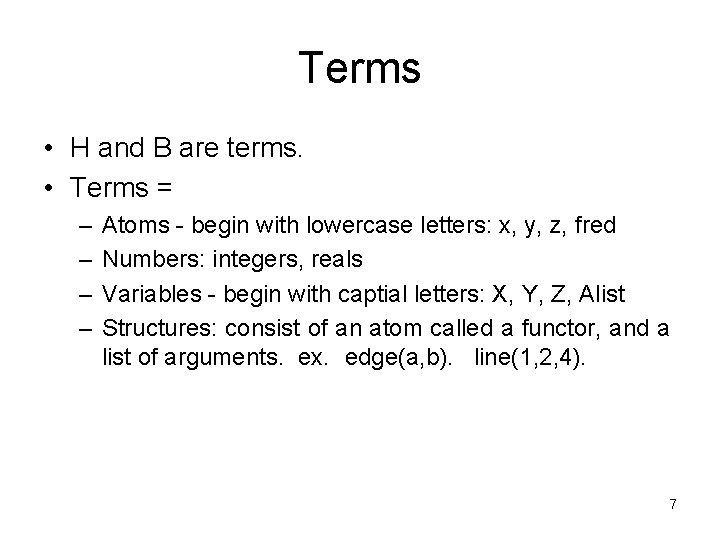Terms • H and B are terms. • Terms = – – Atoms - begin with lowercase letters: x, y, z, fred Numbers: integers, reals Variables - begin with captial letters: X, Y, Z, Alist Structures: consist of an atom called a functor, and a list of arguments. ex. edge(a, b). line(1, 2, 4). 7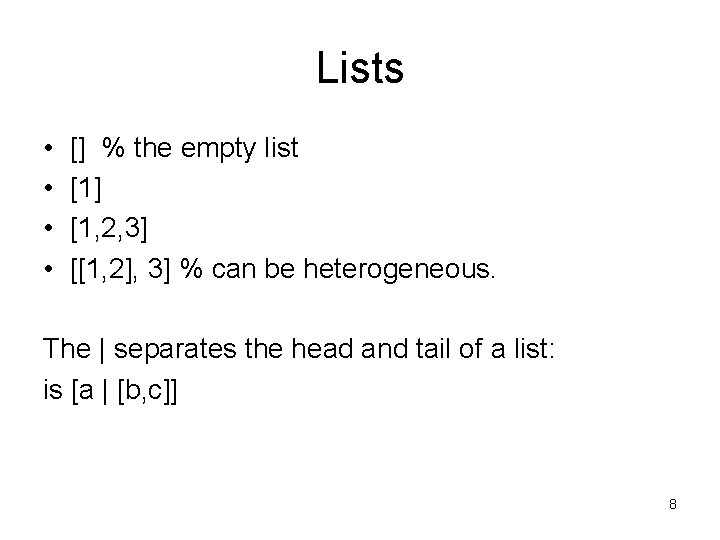Lists • • [] % the empty list  [1, 2, 3] [[1, 2], 3] % can be heterogeneous. The | separates the head and tail of a list: is [a | [b, c]] 8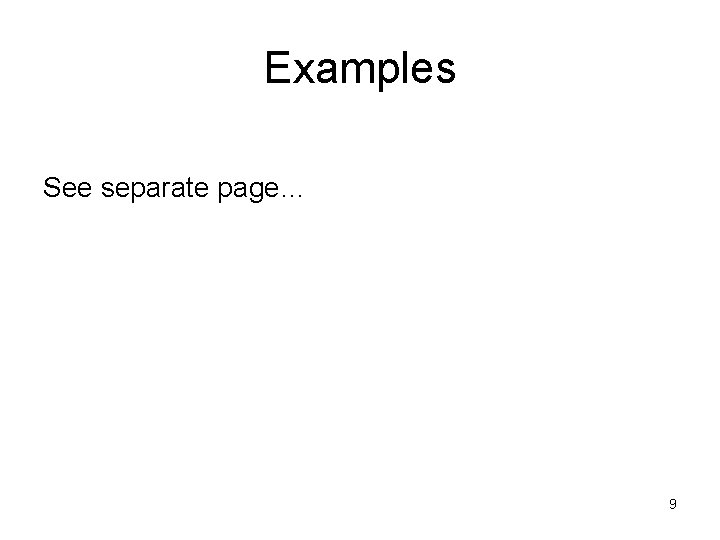Examples See separate page… 9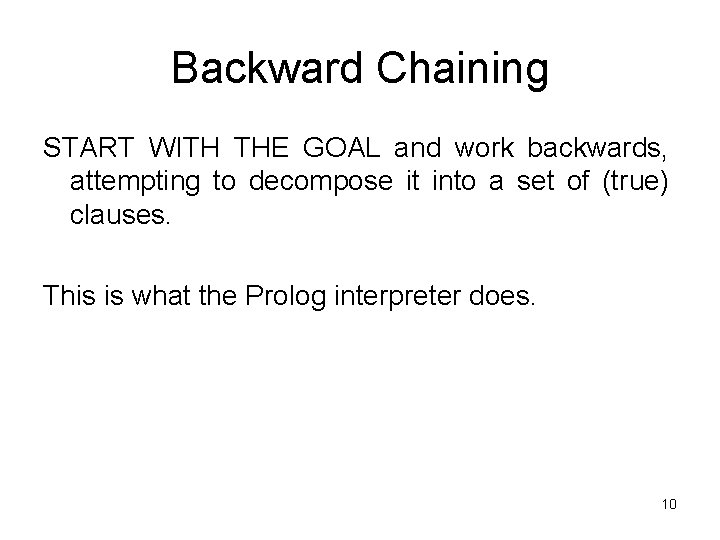Backward Chaining START WITH THE GOAL and work backwards, attempting to decompose it into a set of (true) clauses. This is what the Prolog interpreter does. 10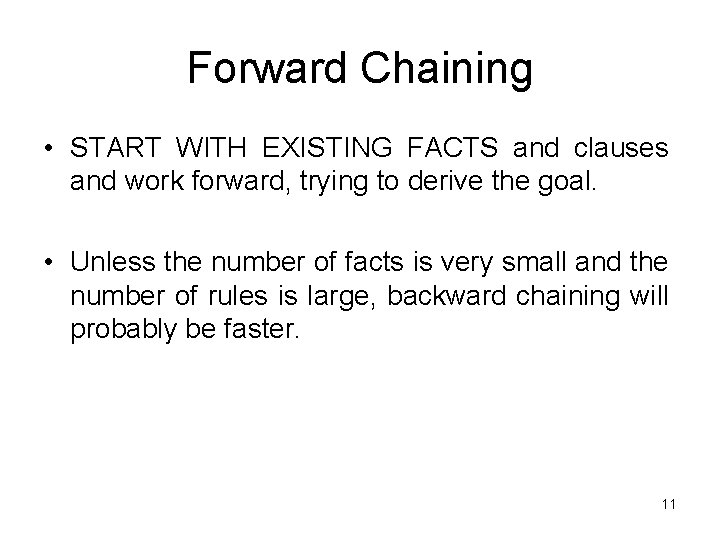Forward Chaining • START WITH EXISTING FACTS and clauses and work forward, trying to derive the goal. • Unless the number of facts is very small and the number of rules is large, backward chaining will probably be faster. 11Searching the database as a tree • DEPTH FIRST - finds a complete sequence of propositions for the first subgoal before working on the others. (what Prolog uses) • BREADTH FIRST - works on all subgoals in parallel. • The implementers of Prolog chose depth first because it can be done with a stack (expected to use fewer memory resources than breadth first). 12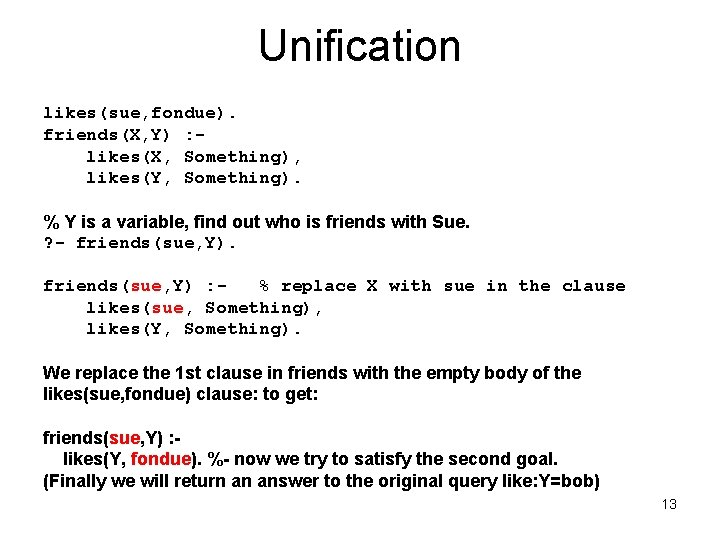Unification likes(sue, fondue). friends(X, Y) : likes(X, Something), likes(Y, Something). % Y is a variable, find out who is friends with Sue. ? - friends(sue, Y) : % replace X with sue in the clause likes(sue, Something), likes(Y, Something). We replace the 1 st clause in friends with the empty body of the likes(sue, fondue) clause: to get: friends(sue, Y) : likes(Y, fondue). %- now we try to satisfy the second goal. (Finally we will return an answer to the original query like: Y=bob) 13Backtracking search 14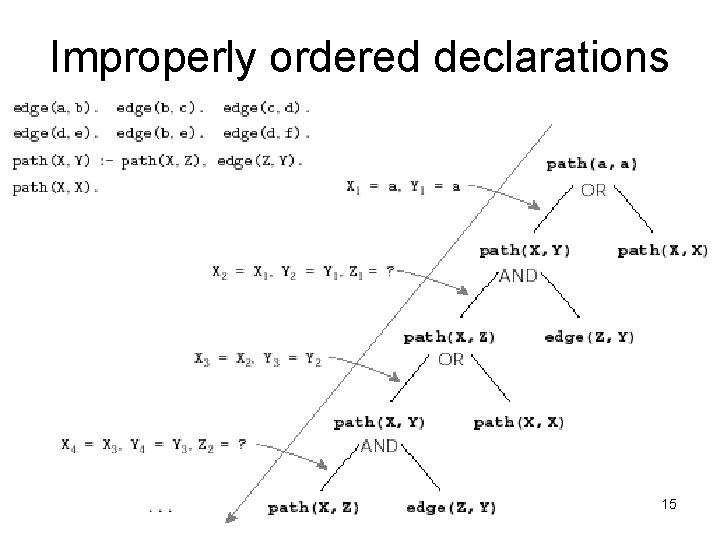Improperly ordered declarations 15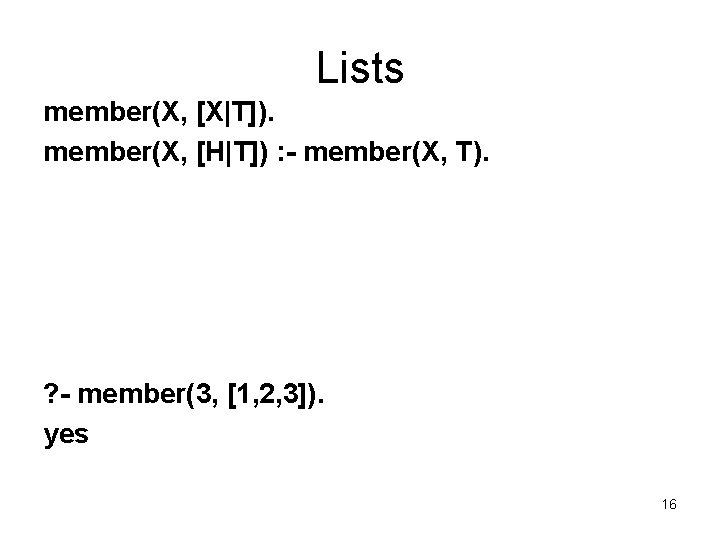Lists member(X, [X|T]). member(X, [H|T]) : - member(X, T). ? - member(3, [1, 2, 3]). yes 16Lists member(X, [X|T]). member(X, [H|T]) : - member(X, T). ? - member(X, [1, 2, 3]). X=1; X=2; X=3; no 17Lists append([], L, L). append([H|T], L, [H|L 2]) : - append(T, L, L 2). | ? - append([1, 2], [3, 4, 5], X). X = [1, 2, 3, 4, 5] 18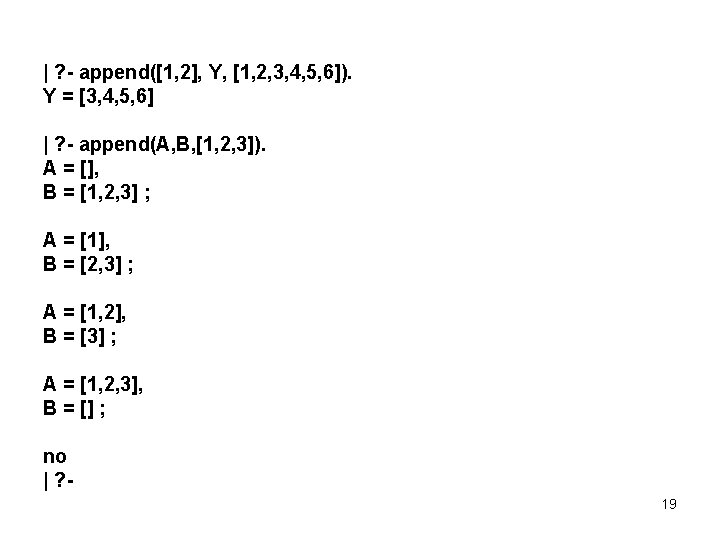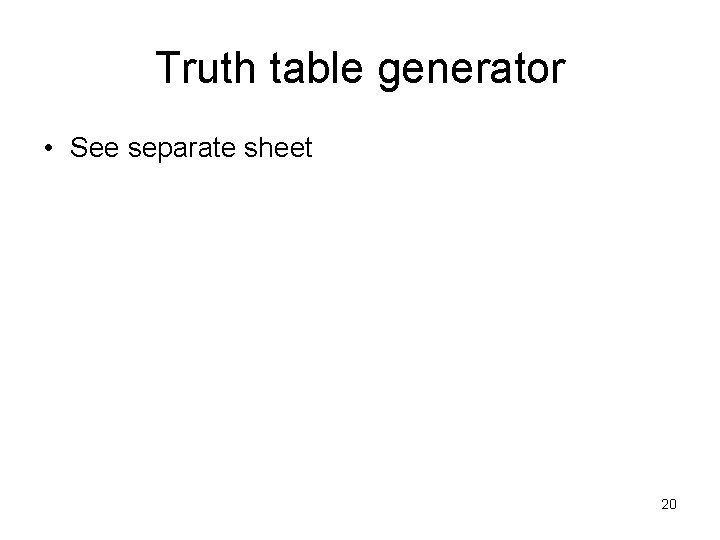Truth table generator • See separate sheet 20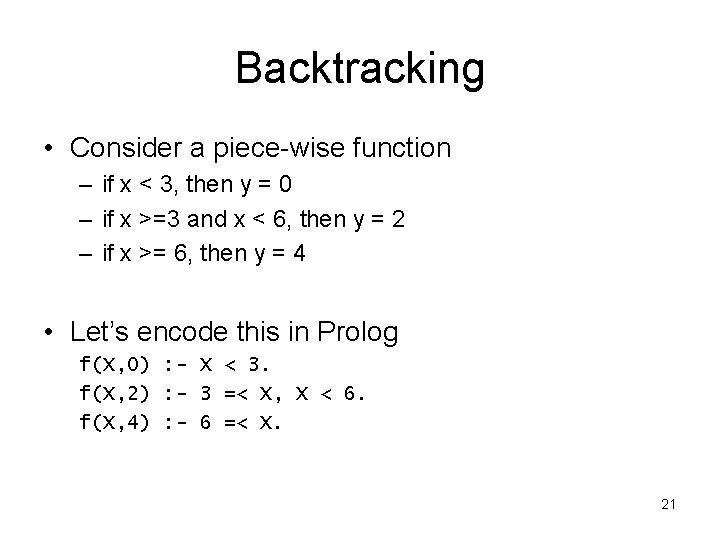Backtracking • Consider a piece-wise function – if x < 3, then y = 0 – if x >=3 and x < 6, then y = 2 – if x >= 6, then y = 4 • Let’s encode this in Prolog f(X, 0) : - X < 3. f(X, 2) : - 3 =< X, X < 6. f(X, 4) : - 6 =< X. 21Backtracking • Let’s encode this in Prolog f(X, 0) : - X < 3. f(X, 2) : - 3 =< X, X < 6. f(X, 4) : - 6 =< X. • Consider ? - f(1, Y), 2<Y. • This matches the f(X, 0) predicate, which succeeds – Y is then instantiated to 0 – The second part (2<Y) causes this query to fail • Prolog then backtracks and tries the other predicates – But if the first one succeeds, the others will always fail! – This, the extra backtracking is unnecessary 22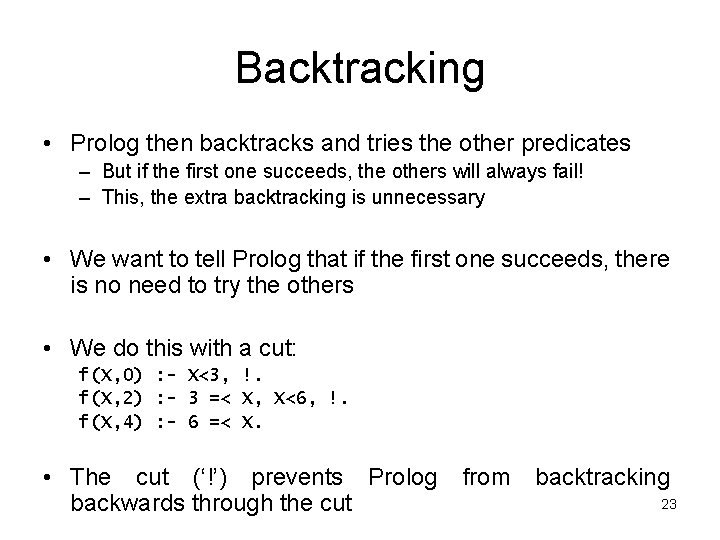Backtracking • Prolog then backtracks and tries the other predicates – But if the first one succeeds, the others will always fail! – This, the extra backtracking is unnecessary • We want to tell Prolog that if the first one succeeds, there is no need to try the others • We do this with a cut: f(X, 0) : - X<3, !. f(X, 2) : - 3 =< X, X<6, !. f(X, 4) : - 6 =< X. • The cut (‘!’) prevents Prolog backwards through the cut from backtracking 23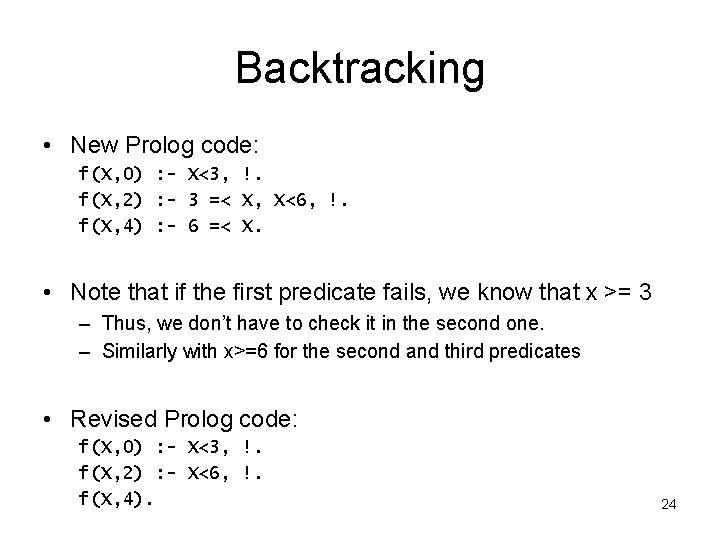Backtracking • New Prolog code: f(X, 0) : - X<3, !. f(X, 2) : - 3 =< X, X<6, !. f(X, 4) : - 6 =< X. • Note that if the first predicate fails, we know that x >= 3 – Thus, we don’t have to check it in the second one. – Similarly with x>=6 for the second and third predicates • Revised Prolog code: f(X, 0) : - X<3, !. f(X, 2) : - X<6, !. f(X, 4). 24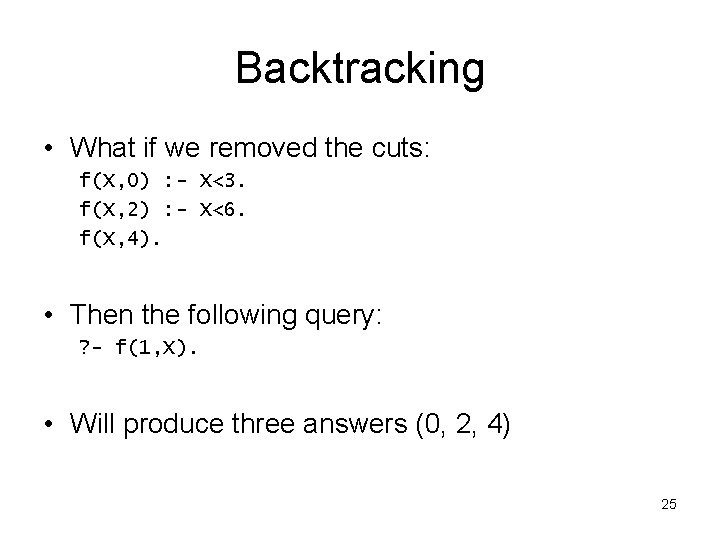Backtracking • What if we removed the cuts: f(X, 0) : - X<3. f(X, 2) : - X<6. f(X, 4). • Then the following query: ? - f(1, X). • Will produce three answers (0, 2, 4) 25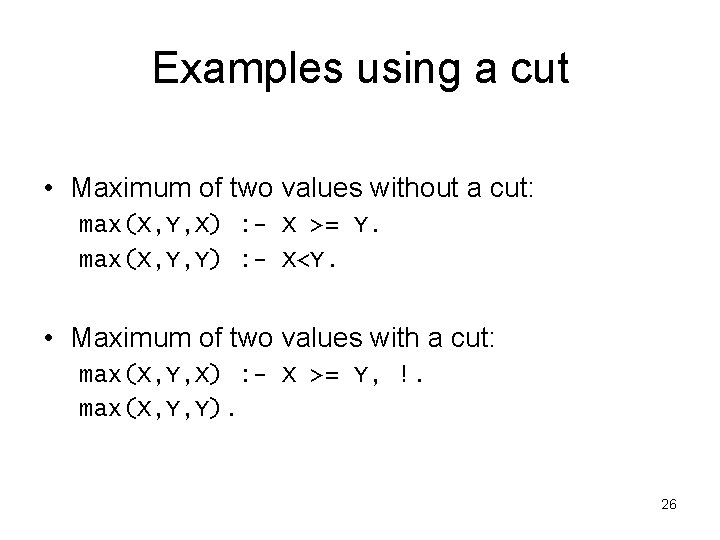Examples using a cut • Maximum of two values without a cut: max(X, Y, X) : - X >= Y. max(X, Y, Y) : - X<Y. • Maximum of two values with a cut: max(X, Y, X) : - X >= Y, !. max(X, Y, Y). 26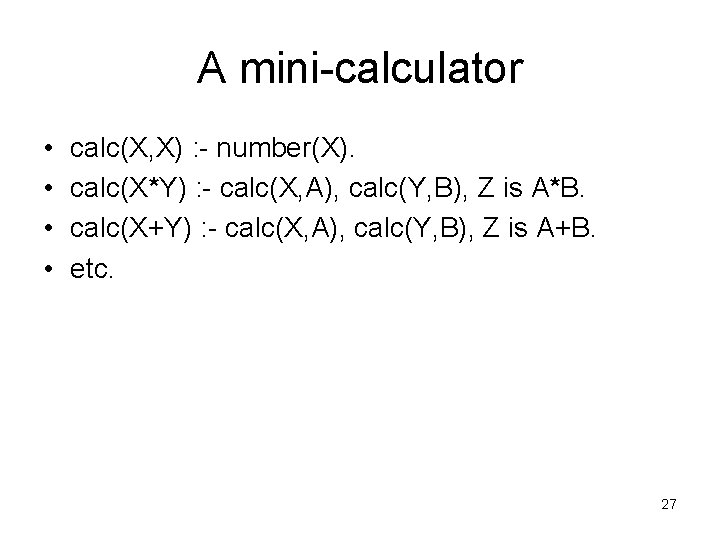A mini-calculator • • calc(X, X) : - number(X). calc(X*Y) : - calc(X, A), calc(Y, B), Z is A*B. calc(X+Y) : - calc(X, A), calc(Y, B), Z is A+B. etc. 27